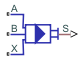# Cartridge Valve Actuator (IL)

Actuator that maintains equilibrium between valve and pilot pressures in an isothermal liquid system

Since R2020a

•Libraries:
Simscape / Fluids / Valve Actuators & Forces

## Description

The Cartridge Valve Actuator (IL) block models an actuator that maintains equilibrium between the valve and pilot-line pressures. The valve between ports A and B remains closed until the pilot spring Spring preload force is surpassed, at which point the piston begins to move. The piston position is output as a physical signal at port S. A schematic of a 4-port cartridge valve actuator is shown below.### Actuator Force Balance

The actuator piston moves to adjust the pressure in the actuator chamber, which maintains equilibrium between the actuator port pressures and pilot line pressures:

`${p}_{A}{A}_{A}+{p}_{B}{A}_{B}={F}_{preload}+{F}_{pilot},$`

where:

• pA and pB are the pressures at ports and B.

• AX is calculated from the Port A to port X area ratio.

• AB is the port B area, ${A}_{X}-{A}_{A}$, when the Number of pressure ports is set to `3`. When the Number of pressure ports is set to `4`, AB is ${A}_{X}-{A}_{A}+{A}_{Y}$.

• Fpreload is the initial spring force in the system.

• Fpilot is ${p}_{X}{A}_{X}$ if Number of pressure ports is set to `3` and ${p}_{X}{A}_{X}+{p}_{Y}{A}_{Y}$ if Number of pressure ports is set to `4`.

### Piston Position

The steady piston displacement is calculated as:

`${x}_{steady}=\frac{F\epsilon }{k}=\frac{{F}_{A}+{F}_{B}-{F}_{pilot}-{F}_{preload}}{k}\epsilon ,$`

where ε is the Opening orientation, which assigns movement in a positive direction (extension) or negative direction (retraction). The dynamic change in piston displacement is:

`${\stackrel{˙}{x}}_{dyn}=\frac{{x}_{steady}-{x}_{dyn}}{\tau },$`

where τ is the Actuator time constant. When ${\stackrel{˙}{x}}_{dyn}=\frac{{x}_{dyn}}{\tau }.$ is less than the Spring preload force, xsteady = 0.

If ${\stackrel{˙}{x}}_{dyn}=\frac{{x}_{dyn}}{\tau }.$ is greater than the sum of the preload force and kxstroke, xsteady = xstroke.

### Opening Dynamics

If opening dynamics are modeled, a lag is introduced to the flow response to the modeled control pressure. pcontrol becomes the dynamic control pressure, pdyn; otherwise, pcontrol is the steady-state pressure. The instantaneous change in dynamic control pressure is calculated based on the Opening time constant, τ:

`${\stackrel{˙}{p}}_{dyn}=\frac{{p}_{control}-{p}_{dyn}}{\tau }.$`

By default, Opening dynamics is set to `Off`.

### Numerically-Smoothed Force

When the actuator is close to full extension or full retraction, you can maintain numerical robustness in your simulation by adjusting the block . A smoothing function is applied to all calculated forces, but primarily influences the simulation at the extremes of the piston motion.

The normalized force is

`$\stackrel{^}{F}=\frac{{F}_{A}+{F}_{B}-{F}_{Preload}-{F}_{Pilot}}{k{x}_{stroke}}.$`

where:

• FA is the force at port A.

• FB is the force at port B.

• FPilot is the force at port X.

If the parameter is nonzero, the block smoothly saturates the normalized force between `0` and `1`.

### Assumptions and Limitations

Internal fluid volumes are not modeled in this block. There is no mass flow rate through ports A, B, X, and Y.

## Ports

### Conserving

expand all

Pressure port associated with the valve inlet.

Pressure port associated with the valve outlet.

Pressure port at poppet end.

Pilot pressure port. To enable this port, set Number of pressure ports to `4`.

### Output

expand all

Poppet position in m, specified as a physical signal.

## Parameters

expand all

Number of pilot pressure ports. Port X measures the pressure at the end of the poppet. Setting this parameter to `4` enables the additional pilot-side port Y.

Cross-sectional area of port A.

Ratio of the inlet port, A, to the pilot pressure port, X. This value is used to calculate the force at port X.

Cross-sectional area of port Y.

#### Dependencies

To enable this parameter, set Number of pressure ports to `4`.

Spring force in the valve due to spring preloading. This parameter is a threshold value which, when added to the pilot pressures at ports X and Y, counterbalances the valve opening due to the pressures at ports A and B.

Spring stiffness constant.

Maximum poppet extension.

Continuous smoothing factor that introduces a layer of gradual change to the piston position when it is near full extension or full retraction. Set this value to a nonzero value less than one to increase the stability of your simulation in these regimes.

Direction of the poppet displacement that opens a connected valve. Setting this parameter to ```Positive displacement opens valve``` indicates poppet extension. Setting the parameter to `Negative displacement opens valve` indicates poppet retraction.

Whether to account for transient effects in the spool position due to actuation. Setting Actuator dynamics to `On` approximates actuator motion by introducing a first-order lag in the spool position. The Actuator time constant also impacts the modeled dynamics.

Constant that captures the time required for the piston to reach steady-state when moving from one position to another. This parameter impacts the modeled actuator dynamics.

#### Dependencies

To enable this parameter, set Actuator dynamics to `On`.

## Version History

Introduced in R2020a